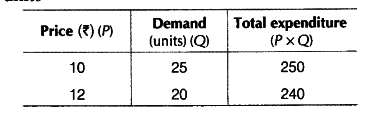# When the price of a good rises from Rs 10 to Rs 12 per unit, its demand falls from 25 units, to 20 units

When the price of a good rises from Rs 10 to Rs 12 per unit, its demand falls from 25 units, to 20 units. What can you say about Price Elasticity of Demand of the good through the 'expenditure approach?

Given, P = Rs 10,P1= Rs 12, Q = 25 units, Q1 = 20 units.As per the expenditure approach, price increases’ and TE decreases. It shows inverse relationship between price and total expenditure. So, it shows that demand is elastic or greater that unity, i.e. {{E}_{d}} > 1.